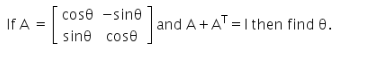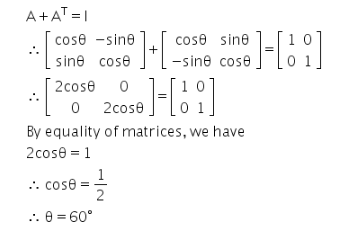# Given below A matrix and A+A^T=I then find θ

Given below A matrix and A+A^T=I then find θ